Next: Auxilliary Eigenfunctions Up: Terrestrial Ocean Tides Previous: Another Useful Lemma

# Basis Eigenfunctions

Suppose that the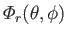are well-behaved solutions of the eigenvalue equation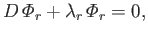(12.230)

subject to the boundary conditions(12.231)

It immediately follows from Equations (12.234) and (12.235) that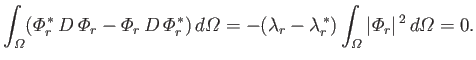(12.232)

Hence, we deduce that the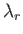are real. It follows that we can choose the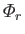to be real functions. Equations (12.234) and (12.235) also yield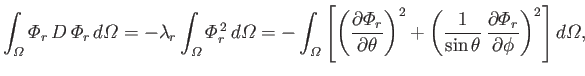(12.233)

which implies that theare positive. Integration of Equation (12.235), subject to the boundary condition (12.236), gives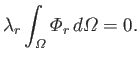(12.234)

Becauseis positive, this implies that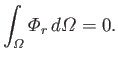(12.235)

Finally, Equations (12.234) and (12.235) yield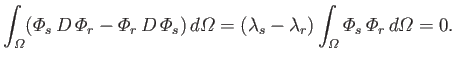(12.236)

It follows that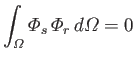(12.237)

if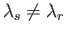. As is well known (Riley 1974), ifand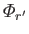are linearly independent solutions of (12.235) corresponding to the same eigenvalue,, then it is always possible to choose linear combinations of them that satisfy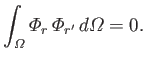(12.238)

This argument can be extended to multiple linearly independent solutions corresponding to the same eigenvalue. Hence, we conclude that it is possible to choose thesuch that they satisfy the orthonormality condition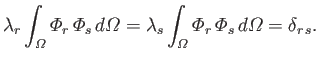(12.239)

Let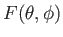be a well-behaved function. Suppose that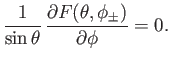(12.240)

We can automatically satisfy the previous boundary condition by writing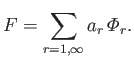(12.241)

(Note thatis undetermined to an arbitrary additive constant which is chosen so as to ensure that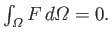) Here,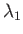is the smallest eigenvalue of Equation (12.235),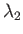the next smallest eigenvalue, and so on. It follows from Equation (12.244) that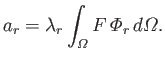(12.242)

Suppose that the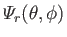are well-behaved solutions of the eigenvalue equation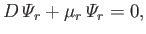(12.243)

subject to the boundary conditions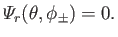(12.244)

Using analogous arguments to those employed previously, we can show that the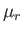are real and positive, and that thecan be chosen so as to satisfy the orthonormality constraint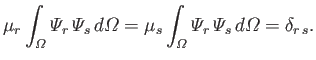(12.245)

Letbe a well-behaved function. Suppose that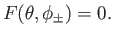(12.246)

We can automatically satisfy the previous boundary condition by writing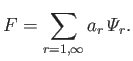(12.247)

It follows from Equation (12.250) that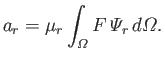(12.248)Next: Auxilliary Eigenfunctions Up: Terrestrial Ocean Tides Previous: Another Useful Lemma
Richard Fitzpatrick 2016-03-31